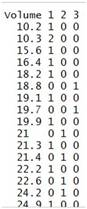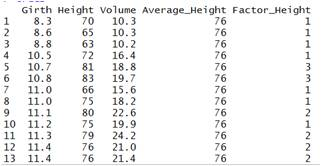Thursday, October 22, 2015

## Before we get started …

• install the package "datasets" and "data.table", and load it in
require(datasets)
require(data.table)
## Loading required package: data.table
## Warning: package 'data.table' was built under R version 3.1.3
• We would use "sleep" in this lab session
• Before we get started, type the following:
dt <- data.table(sleep)

## Question 1

What are the differences between data.frame() and data.table()?

## Answer 1

# - data.table can extract rows without commas
# - data.table allow multiple functions to compute on data

## Question 2

Extract the second and third rows in "sleep". (How to do so using data.frame() and data.table()?)

## Answer 2

# data.frame()
sleep[2:3,]
##   extra group ID
## 2  -1.6     1  2
## 3  -0.2     1  3
# data.table()
dt[2:3]
##    extra group ID
## 1:  -1.6     1  2
## 2:  -0.2     1  3

## Question 3

Extract the second and third columns in "sleep". (How to do so using data.frame() and data.table()?)

## Answer 3

# data.frame()
sleep[,2:3]

# data.table()
dt[,.(group,ID)]

## Question 4

What is the sum of the first column in "sleep"? (How to do so using data.frame() and data.table()?)

## Answer 4

# data.frame()
sum(sleep$extra) ##  30.8 # data.table() dt[,.(total = sum(sleep$extra))] 
##    total
## 1:  30.8

## Question 6

Explore dataset "trees". How many rows and columns?

## Answer 6

dim(trees)
##  31  3

## Question 7

In "trees", extract rows that have Girth larger than 12.0 (exclude) and Height larger than 80 (include).

## Answer 7

attach(trees)
trees[Girth > 12.0 & Height >= 80, ]
##    Girth Height Volume
## 17  12.9     85   33.8
## 18  13.3     86   27.4
## 22  14.2     80   31.7
## 26  17.3     81   55.4
## 27  17.5     82   55.7
## 28  17.9     80   58.3
## 29  18.0     80   51.5
## 30  18.0     80   51.0
## 31  20.6     87   77.0
detach(trees)

## Before we get started …

We would use "trees" dataset in this exercise.

## Exercises

• What is the maximum value of Height?
• Group the data "trees" by Volume.
• Create a new column Average_Height, and put the value of average height to the column.
• Extract rows that have Volume not equal to 10.3.
• Create a new column Factor_Height. Change the numeric type of Height into factors. And for values that are lower than 75 (include), label as level "1"; values that range from 76-80, as level "2"; and values that are higher than 81, assigned as level "3".
• Count the occurrences of Volume by Factor_Height.
• So, what is a factor? What can we do with it?

## Exercises

• For question (f), the final output should look like the following:• The final output of your dataframe should look like the following:## Answers

attach(trees)
max(Height)

trees[order(Volume),]

trees$Average_Height <- mean(Height) trees[Volume !=10.3,] factor_height <- factor(Height) levels(factor_height) <- c(rep(1,10),rep(2,5), rep(3,6)) trees$Factor_Height <- factor_height

table(Volume, trees\$Factor_Height)
detach(trees)

## A factor could be used to help doing data categorization.